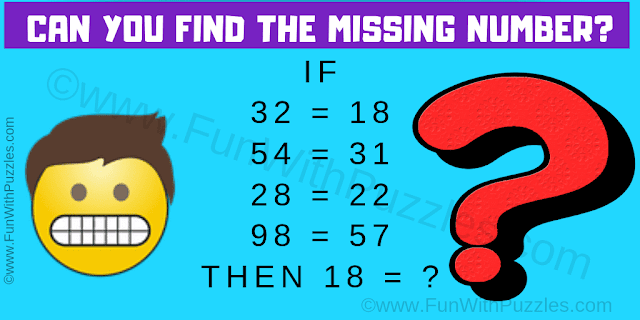## Friday, October 4, 2019

This is a mind cracking maths logic puzzle question which will shake your brain. In this maths logic question, your challenge is to find the value of the missing number. You need to first decipher the logical reasoning used in the given logical number equations. Apply the same logical reasoning to the last number equation to find the value of the missing number which replaces the question mark. If you can crack this mind cracking logic question, then you are a genius. Post your answer in the comments.Can you crack this mind cracking maths logic question?
The answer to this "Mind Cracking Maths Logic Question", can be viewed by clicking on the answer button.

1.1.